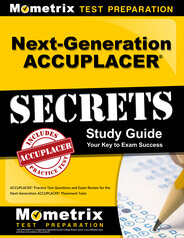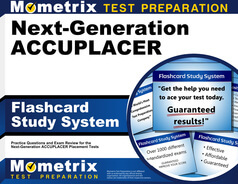Next Generation ACCUPLACER Arithmetic, Quantitative Reasoning, Algebra and Statistics Practice Test

The Next Generation ACCUPLACER arithmetic, quantitative reasoning, algebra and statistics subtests are computer-adaptive tests comprised of 20 questions of varying complexities. Since the ACCUPLACER math tests are computer adaptive, they will automatically select an appropriate difficulty level for the questions you are presented based on your previous responses; this process serves to accurately represent your individual skill and knowledge level to appropriately place you into classes. These tests are appropriate for students entering non-STEM fields of study, or those whose majors are undecided.

 Next-Gen ACCUPLACER Test Next-Gen ACCUPLACER Study Guide Next-Gen ACCUPLACER Flashcards

Calculator Use

Handheld calculators are not permitted for use on the ACCUPLACER math tests and will not be allowed into the test center. In the event a question is configured to allow for calculator use, you will be given access to one on the computer through an icon on the screen.

How to Make an Appointment

The ACCUPLACER tests are administered by College Board. If you would like to schedule an appointment, you should start by visiting with your college advisor or counselor. If your institution is located in another state, there may be remote testing options available to take your ACCUPLACER test. Your advisor or counselor will be able to guide you further on the necessary steps to receive permission for remote testing and schedule your appointment.

Next Generation ACCUPLACER Arithmetic Subtest

You will be presented with 20 questions on the ACCUPLACER arithmetic subtest. The questions will assess your comprehension of fundamental arithmetic concepts, including the following knowledge and skills categories:

• Decimal Operations (3-5 questions / 15-25%)
• Addition, Division, Multiplication and Subtraction of Decimal Numbers
• Applying Operations to Real-Life Contexts
• Estimation and Rounding
• Order of Operations
• Fraction Operations (3-5 questions / 15-25%)
• Addition, Division, Multiplication and Subtraction of Fractions and Mixed Numbers
• Applying Operations to Real-Life Contexts
• Estimation and Rounding
• Order of Operations
• Number Comparisons and Equivalents (3-5 questions / 15-25%)
• Comparisons of Differently Formatted Values by Ordering
• Evaluation of Equivalent Number Statements
• Using Equality/Inequality Symbol Notation
• Using the Number Line
• Percent (3-5 questions / 15-25%)
• Applying Percent to Real-Life Contexts
• Calculation with Percent (with or without a context)
• Determining the Percent of a Number
• Percent Decrease
• Percent Increase
• Whole Number Operations (3-5 questions / 15-25%)
• Addition, Division, Multiplication and Subtraction of Whole Numbers
• Applying Operations to Real-Life Contexts
• Estimation and Rounding
• Order of Operations

Next Generation ACCUPLACER Quantitative Reasoning, Algebra and Statistics (QAS) Subtest

When you take the Next Generation ACCUPLACER quantitative reasoning, algebra and statistics (QAS) subtest, you will be asked to respond to 20 questions designed to assess your level of comprehension of the following knowledge and skills:

• Algebraic Expressions (2-3 questions / 10-15%)
• Creating and Evaluating Expressions to Represent Situations
• Identify Equivalent Expressions
• Using Properties of Operations to Combine Like Terms
• Descriptive Statistics (1-3 questions / 5-15%)
• Calculating Measures of Center
• Describing Shape and Spread of a Sample Set
• Interpreting Graphical Displays of Data (histograms, box plots and scatterplots)
• Exponents (2-3 questions / 10-15%)
• Applying Scientific Notation
• Calculating with Exponents, Radicals and Fractional Exponents
• Geometry Concepts for Algebra I (1-2 questions 5-10%)
• Creating Expressions for Area, Perimeter and Volume
• Evaluating Basic Geometric Transformations
• Using Distance Formula and Pythagorean Theorem
• Geometry Concepts for Pre-Algebra (1-2 questions / 5-10%)
• Circle Area and Circumference
• Determining Area and Perimeter
• Volume of Prisms
• Linear Applications and Graphs (2-4 questions / 10-20%)
• Applying Linear Equations to Real-Life Contacts
• Graphing Linear Equations in Two Variables
• Linear Inequalities
• Parallel and Perpendicular Lines
• Systems of Equations
• Using Elementary Linear Functions to Describe Relationships
• Linear Equations (2-4 questions / 10-20%)
• Creating Linear Equations in One or Two Variables
• Simplifying Linear Equations and Inequalities
• Solving Linear Equations
• Solving Systems of Two Linear Equations
• Probability and Sets (1-3 questions / 5-15%)
• Calculating Probability (simple, compound and conditional)
• Defining Sample Spaces and Events Using Set Notation
• Ratio and Proportional Relationships (3-4 questions / 15-20%)
• Calculating with Rates, Ratios and Proportions (with or without a context)
• Usage of Absolute Value
• Rational Numbers (1-3 questions / 5-15%)
• Calculating and Applying Rational Numbers (with or without a context)
• Usage of Absolute Value

Preparing for the ACCUPLACER Math Test

As you prepare to take one of the ACCUPLACER math subtests, take advantage of the resources available to you in order to perform at your best. Mometrix’s free ACCUPLACER test prep and study resources include ACCUPLACER practice tests and ACCUPLACER study guides to review critical concepts before test day.

Next Generation ACCUPLACER Study Guide

Next Generation ACCUPLACER FlashcardsACCUPLACER Next Generation Test

by Mometrix Test Preparation | Last Updated: May 13, 2019